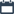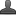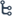## Friday, October 16, 2020

•October 16, 2020
••, , ,
•1 comment

## IIT JEE 7th class Mathematics Introduction to Algebra Notes, Definition, concept, important points

ALGEBRA

Hi friends and my dear students! In this post, I have covered class -7 Introduction to Algebra (Foundation IIT) important points After Reading Mathematics Algebra (7th class ) IIT Notes With important points, please do share it with your friends. You can Learn Maths for All Classes here.

Variable: A letter symbol which can take any value of a certain set.

Eg: a, x , y., etc.,

Constants: Quantities which have only one fixed value.

Eg : 2, 5, sometimes letters are indicated as constants.

Term: Numerical numbers alone or literal numbers alone or their combinations by operation of multiplication. Eg: 2a, 3x.

Exponent & Base: If a¹0 then a x a x a....  m times = am, here ‘a’ is called base and ‘m’ is called exponent. ‘am ’ can be read as mth power of ‘a’.

Eg: In x2, x is base and 2 is exponent or index

Algebraic Expression: A combination of terms by the Mathematical operations. Eg: 3x–2y, x+2, 5x2

Coefficient: In a product containing two or more than two factors, each factor is called the coefficient of the product of other factors.

Eg: In 2a, 2 is coefficient of ‘a’ which is called as numerical coefficient. ‘a’ is called literal coefficient of 2.

Like and Unlike Terms : Terms which contain the same variable or literal factor are called like terms, othe rwise they are called unlike terms.

Eg: x2,5x2,7x2 are like terms. 5x,7 x2,3y are unlike terms.

Value of an Algebraic Expression: By substituting numerical values in place of variables gives the value of Algebraic expression. Eg: In 3x+4, when x=2 then 3x+4=6+4=10   Types of

Algebraic expressions:

1. Monomial: Having one term. Eg: 2x, 3y2, 5

2. Binomial : Having two terms. Eg: 3x+2y, x2+2

3. Trinomial: Having three terms. Eg: a+b+c

4. Multinomial : Having more than three terms Eg: ax + by + c + 2

Polynomial: An algebraic expression in which the variables involved have only non – negative integral powers, is called a polynomial.

Eg : 1.  2/ 3 x 3- 3 /4x2  is a polynomial in variable x where a  1/2 x 3 -3x2+5x1/2+x-1 is not a polynomial, because it contains a term 5 x 1/2 which contains 1/2 as the power of variable x, which is not a non – negative integer.

2. 1 + 2x + 3y, 4x2y – 5y  etc., are polynomials No polynomial should contain a term having a variable in its denominator

Eg: x + 1/x  , x2+2x+1/x2 are not

Polynomial in one variable: An algebraic expression involving only one variable in which the powers of the variable are non – negative integers, is a polynomial in that variable.

Eg : 3x + 7 is a polynomial in x,

2y2 – 5y + 1 is a polynomial in y

Polynomials in two or more variables: An

algebraic expression involving two or more variable with non – negative integral powers is called a polynomial in these variables.

Eg : x + y + xy is a polynomial in x & y,

a2 + ab2 + 3ab + 5 is a polynomial in a & b

Difference between multinomial and polynomial:

1. A  polynomial is concerned with positive integral powers of the variable involved in the expression and not with the number of terms in the expression.

2.A multinomial is concerned with number of terms in the expression and not with the powers of the variable in the expression. Thus

all polynomials are multinomials, But all

multinomials need not be polynomials.

Eg:3x+4/xis a multinomial but not a polynomial

More definitions: If the coefficients of the terms in a polynomial are integers, it is called a polynomial with integral coefficient. Eg : z 2 + 4z2 + 3z – 6, here coefficients of terms 1, 4, 3, -6 are integers If the coefficients of the terms in a polynomial are rationals, it is called a polynomial with rational

1/3 x2y+4/5xy2+xyz here coefficients of

Terms 1/3,4/5,1are rationals.

Also Check

## Trigonometry Do This & Try this solution

SSC(10th class) Trigonometry Exercise - 11.1 Solutions

An algebraic expression of the form a + bx + cx2 +……….is called a polynomial in a single variable x. Here a, b, c ….. are rationals  such that atleast one of them is non – zero. If all the coefficients a, b, c, are zero then it is called a zero polynomial. Since a, b, c…. are zeros then polynomial is 0 + 0. x  + 0. x2 +…..= 0. Hence 0 is called zero polynomial.

Zero of a polynomial: The number for which the value of a polynomial is zero, is called zero of the polynomial.

Eg: Find the zero of the polynomial ax + b

Sol: ax + b = 0

ax = -b

ax = -b

x = -b/a

So zero of the polynomial ax + b is -b/a

Difference between zero polynomial and

zero of the polynomial :

Zero polynomial is one type of polynomial where as zero of a

polynomial is a number at which the value of the polynomial is zero.

Degree of a term in a polynomial : The degree of any term of a polynomial is the sum of the

powers of all variables in that term.

Eg : 1. In 3x2y4z6, Only term is 3x2y4z6

Its degree is 2 + 4 + 6 =12

Eg : 2. In x + y + xy, terms are x, y,. xy

Their degrees are1, 1, 2

Eg :3. In 5x3 + 3x2 + 4x – 8, terms are 5x3, 3x2,4x and - 8 Their degree is 3, 2, 1, 0

Note that: Degree of a constant term is zero

since it involves no variable

Eg : 5 = 5. x0 so degree of 5 is 0.

Degree of the polynomial : The degree of the highest degree term in a polynomial is called the Degree of the polynomial.

Eg :

1. Degree of x + 2y + x y is 2

2. Degree of 3x2y4z6 is 12

3. Degree of 5x3 + 3x2 + 4x – 8 is 3

4. Degree of a2b + ab2 + 3ab + 5 is 3

5. Degree of y + z is 1

Note that :

1. Degree of zero polynomial is not defined

2. Degree of a non zero constant polynomial is zero

Linear polynomial: A polynomial of degree‘1’ is called a linear polynomial.

Eg : 3+5x

Linear polynomial.General form of linear polynomial in

variable ‘x’ is a x + b (a ≠0)

Quadratic polynomial:A polynomial of degree ‘2’ is called Quadratic polynomial.5x2 + 3x+ 4 is ax2 + bx+ c (a0)

Variable ‘x’ is ax2 + bx+ c (a≠0)

Cubic Polynomial :A polynomial of degree‘3’ is called cubic polynomial

5x3+ 3x2+ 4x+1 is a Cubic polynomial

General form of a cubic polynomial in

variable x, ax3+ bx2+ cx+ d (a≠0)

Eg: 5x4+ 5x3+ 3x2+ 4x+1  are bi quadratic polynomials

General  Form of  a Bi Quadratic polynomials in variable ‘x’ is ax4+bx3+cx2+dx+e (a≠0)

nth degree polynomial :axn + bxn-1+ cxn-2 +…+ px + q(a≠0) is called a polynomial of degree ‘n’ in one variable in x

Where a, b, c, ……… p, q are complex numbers.

[Here the numerical coefficient of highest degree term is called leading coefficient of that polynomial]

Formulae :

1. (a + b) 2 = a2 + 2ab + b2

2. (a - b) 2 = a2 - 2ab + b2

3. (a + b) (a - b) =  a2 - b2

4. (a + b)3 = a3 + 3a2b + 3a b2 + b3 (or)

a3 + 3ab (a + b ) + b3

5. (a- b)3 = a3 - 3 a2b + 3a b2 - b3 (or)

= a3 - 3ab (a - b) - b3

6. (a+ b+ c) 2 =  a2+ b2  + c2 + 2ab + 2bc + 2ca

7. a3 + b3 = (a + b) (a2- ab + b2 )

8. a3 - b3 = (a - b) ( a2 + ab + b2 )

9. (a + b)2 - (a- b)2 = 4ab

10. (a + b)2 + (a- b)2 = 2a2 + 2b2

(Or)2( a2 + b2 )

#### 1 comment:

1.It's very nice of you to share your knowledge through posts. I love to read stories about your experiences. They're very useful and interesting. I am excited to read the next posts. I'm so grateful for all that you've done. Keep plugging. Many viewers like me fancy your writing. Thank you for sharing precious information with us. Best Maths Olympiad service provider